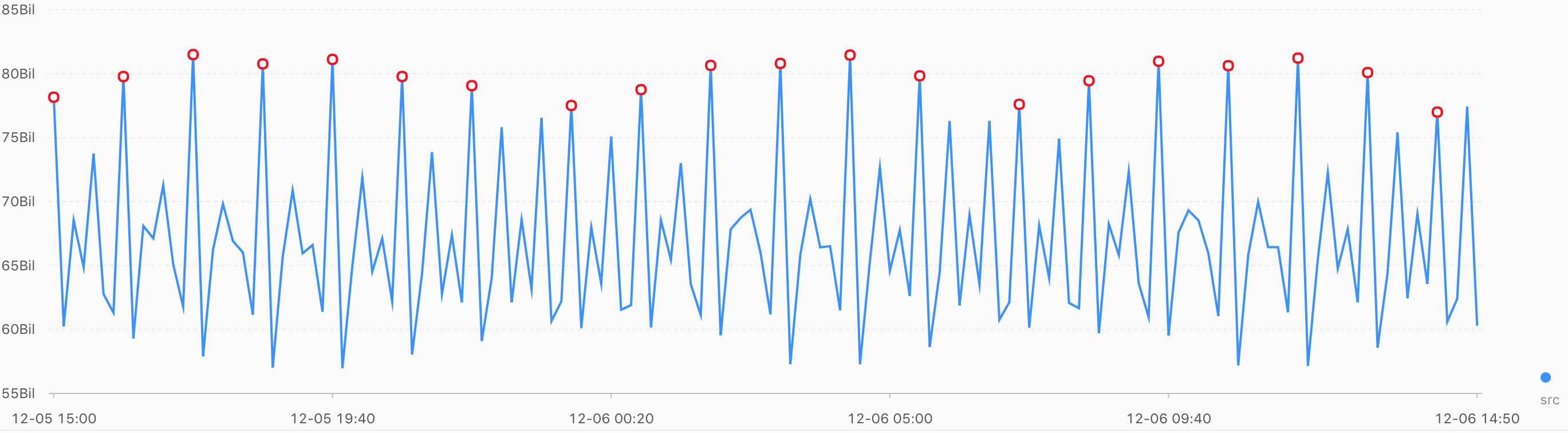This topic describes how to use maximum value detection functions to find the locally maximum value of time series data in a specified window.

## ts_find_peaks

Function format:
``select ts_find_peaks(x, y, winSize)``
The following table lists the parameters of the function format.
Parameter Description Value
x The time sequence. Points in time are sorted in ascending order along the horizontal axis. Each point in time is a Unix timestamp. Unit: seconds.
y The sequence of numeric data corresponding to each specified point in time. N/A.
winSize The minimum length of the detection window. The value of the parameter is of the long data type, ranging from 1 to the length of time series data. We recommend that you set this parameter to one tenth of the actual data length.
Example:
• The query statement is as follows:
``* and h : nu2h05202.nu8 and m: NET |  select ts_find_peaks(stamp, value, 30) from (select __time__ - __time__ % 10 as stamp, avg(v) as value from log GROUP  BY  stamp order by stamp)``
• The following figure shows the output result.The following table lists the display items.
Display item Description
Horizontal axis unixtime The timestamp of time series data. Unit: seconds. Example: 1537071480.
Vertical axis src The raw data. Example: 1956092.7647745228.
peak_flag Indicates whether the numeric value at the time point is the maximum value. Valid values:
• 1.0: The numeric value at the time point is the maximum value.
• 0.0: The numeric value at the time point is not the maximum value.